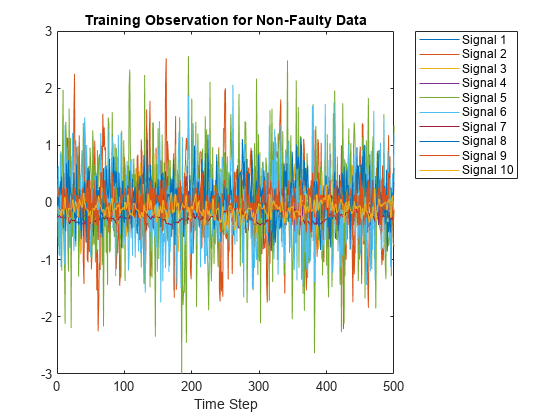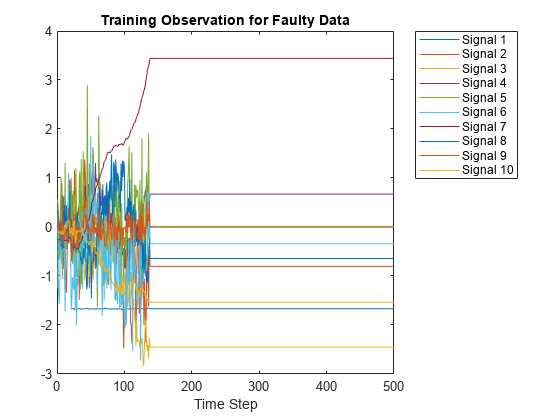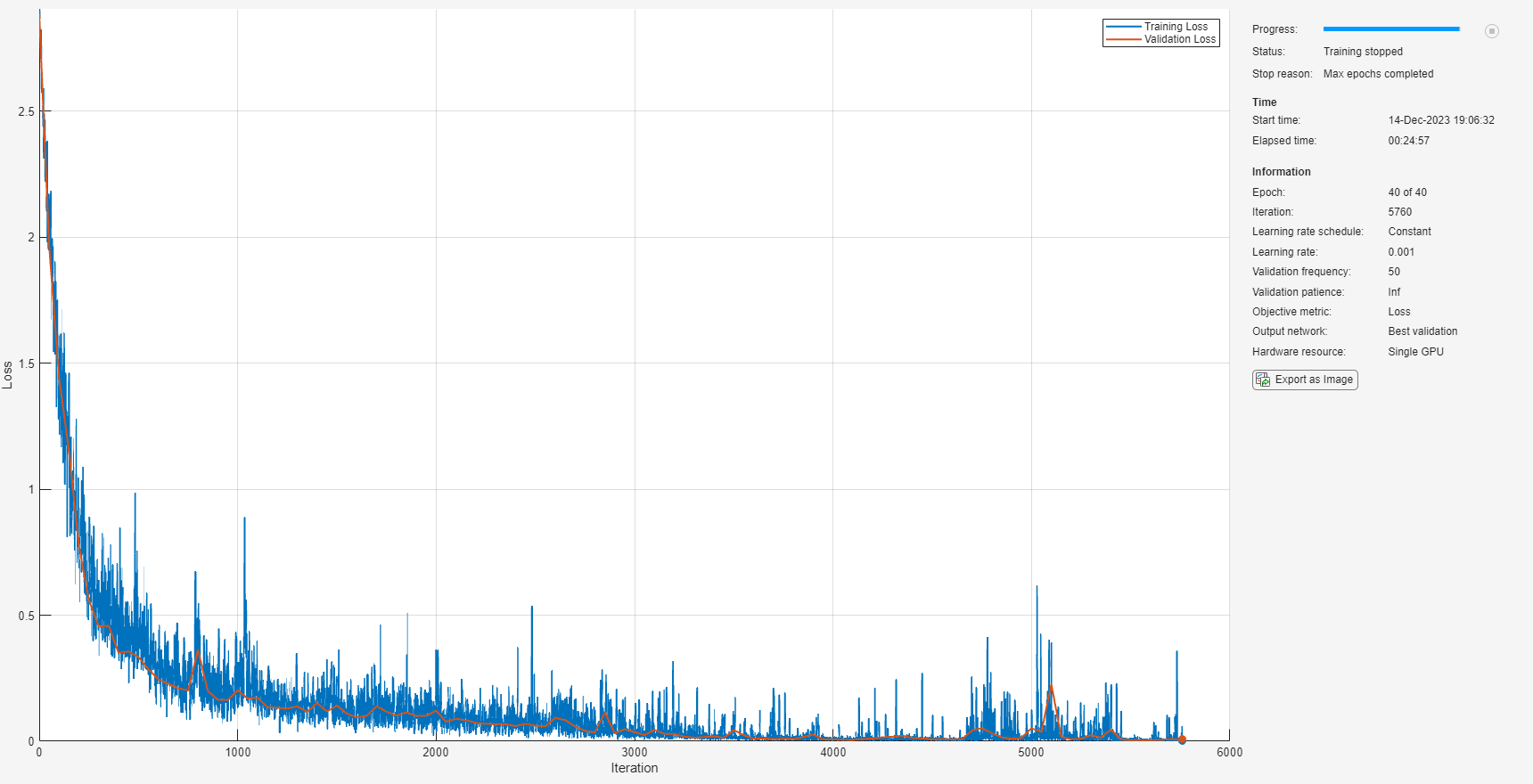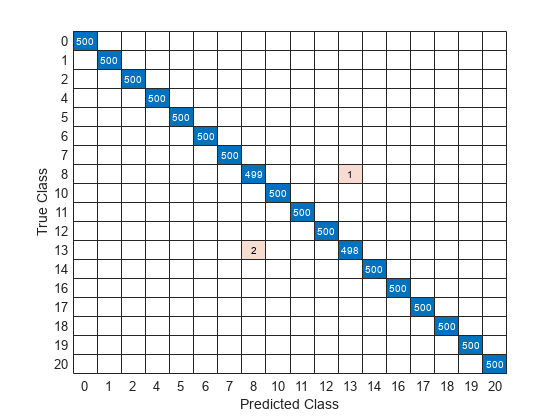# Chemical Process Fault Detection Using Deep Learning

This example shows how to use simulation data to train a neural network that can detect faults in a chemical process. The network detects the faults in the simulated process with high accuracy. The typical workflow is as follows:

1. Preprocess the data

2. Design the layer architecture

3. Train the network

4. Perform validation

5. Test the network

This example uses MATLAB-formatted files converted by MathWorks® from the Tennessee Eastman Process (TEP) simulation data . These files are available at the MathWorks support files site. See the disclaimer.

The data set consists of four components — fault-free training, fault-free testing, faulty training, and faulty testing. Download each file separately.

```url = 'https://www.mathworks.com/supportfiles/predmaint/chemical-process-fault-detection-data/faultytesting.mat'; websave('faultytesting.mat',url); url = 'https://www.mathworks.com/supportfiles/predmaint/chemical-process-fault-detection-data/faultytraining.mat'; websave('faultytraining.mat',url); url = 'https://www.mathworks.com/supportfiles/predmaint/chemical-process-fault-detection-data/faultfreetesting.mat'; websave('faultfreetesting.mat',url); url = 'https://www.mathworks.com/supportfiles/predmaint/chemical-process-fault-detection-data/faultfreetraining.mat'; websave('faultfreetraining.mat',url);```

```load('faultfreetesting.mat'); load('faultfreetraining.mat'); load('faultytesting.mat'); load('faultytraining.mat');```

Each component contains data from simulations that were run for every permutation of two parameters:

• Fault Number — For faulty data sets, an integer value from 1 to 20 that represents a different simulated fault. For fault-free data sets, a value of 0.

• Simulation run — For all data sets, integer values from 1 to 500, where each value represents a unique random generator state for the simulation.

The length of each simulation was dependent on the data set. All simulations were sampled every three minutes.

• Training data sets contain 500 time samples from 25 hours of simulation.

• Testing data sets contain 960 time samples from 48 hours of simulation.

Each data frame has the following variables in its columns:

• Column 1 (`faultNumber`) indicates the fault type, which varies from 0 through 20. A fault number 0 means fault-free while fault numbers 1 to 20 represent different fault types in the TEP.

• Column 2 (`simulationRun`) indicates the number of times the TEP simulation ran to obtain complete data. In the training and test data sets, the number of runs varies from 1 to 500 for all fault numbers. Every `simulationRun` value represents a different random generator state for the simulation.

• Column 3 (`sample`) indicates the number of times TEP variables were recorded per simulation. The number varies from 1 to 500 for the training data sets and from 1 to 960 for the testing data sets. The TEP variables (columns 4 to 55) were sampled every 3 minutes for a duration of 25 hours and 48 hours for the training and testing data sets respectively.

• Columns 4–44 (`xmeas_1` through `xmeas_41`) contain the measured variables of the TEP.

• Columns 45–55 (`xmv_1` through `xmv_11`) contain the manipulated variables of the TEP.

Examine subsections of two of the files.

`head(faultfreetraining,4) `
```ans=4×55 table faultNumber simulationRun sample xmeas_1 xmeas_2 xmeas_3 xmeas_4 xmeas_5 xmeas_6 xmeas_7 xmeas_8 xmeas_9 xmeas_10 xmeas_11 xmeas_12 xmeas_13 xmeas_14 xmeas_15 xmeas_16 xmeas_17 xmeas_18 xmeas_19 xmeas_20 xmeas_21 xmeas_22 xmeas_23 xmeas_24 xmeas_25 xmeas_26 xmeas_27 xmeas_28 xmeas_29 xmeas_30 xmeas_31 xmeas_32 xmeas_33 xmeas_34 xmeas_35 xmeas_36 xmeas_37 xmeas_38 xmeas_39 xmeas_40 xmeas_41 xmv_1 xmv_2 xmv_3 xmv_4 xmv_5 xmv_6 xmv_7 xmv_8 xmv_9 xmv_10 xmv_11 ___________ _____________ ______ _______ _______ _______ _______ _______ _______ _______ _______ _______ ________ ________ ________ ________ ________ ________ ________ ________ ________ ________ ________ ________ ________ ________ ________ ________ ________ ________ ________ ________ ________ ________ ________ ________ ________ ________ ________ ________ ________ ________ ________ ________ ______ ______ ______ ______ ______ ______ ______ ______ ______ ______ ______ 0 1 1 0.25038 3674 4529 9.232 26.889 42.402 2704.3 74.863 120.41 0.33818 80.044 51.435 2632.9 25.029 50.528 3101.1 22.819 65.732 229.61 341.22 94.64 77.047 32.188 8.8933 26.383 6.882 18.776 1.6567 32.958 13.823 23.978 1.2565 18.579 2.2633 4.8436 2.2986 0.017866 0.8357 0.098577 53.724 43.828 62.881 53.744 24.657 62.544 22.137 39.935 42.323 47.757 47.51 41.258 18.447 0 1 2 0.25109 3659.4 4556.6 9.4264 26.721 42.576 2705 75 120.41 0.3362 80.078 50.154 2633.8 24.419 48.772 3102 23.333 65.716 230.54 341.3 94.595 77.434 32.188 8.8933 26.383 6.882 18.776 1.6567 32.958 13.823 23.978 1.2565 18.579 2.2633 4.8436 2.2986 0.017866 0.8357 0.098577 53.724 43.828 63.132 53.414 24.588 59.259 22.084 40.176 38.554 43.692 47.427 41.359 17.194 0 1 3 0.25038 3660.3 4477.8 9.4426 26.875 42.07 2706.2 74.771 120.42 0.33563 80.22 50.302 2635.5 25.244 50.071 3103.5 21.924 65.732 230.08 341.38 94.605 77.466 31.767 8.7694 26.095 6.8259 18.961 1.6292 32.985 13.742 23.897 1.3001 18.765 2.2602 4.8543 2.39 0.017866 0.8357 0.098577 53.724 43.828 63.117 54.357 24.666 61.275 22.38 40.244 38.99 46.699 47.468 41.199 20.53 0 1 4 0.24977 3661.3 4512.1 9.4776 26.758 42.063 2707.2 75.224 120.39 0.33553 80.305 49.99 2635.6 23.268 50.435 3102.8 22.948 65.781 227.91 341.71 94.473 77.443 31.767 8.7694 26.095 6.8259 18.961 1.6292 32.985 13.742 23.897 1.3001 18.765 2.2602 4.8543 2.39 0.017866 0.8357 0.098577 53.724 43.828 63.1 53.946 24.725 59.856 22.277 40.257 38.072 47.541 47.658 41.643 18.089 ```
`head(faultytraining,4) `
```ans=4×55 table faultNumber simulationRun sample xmeas_1 xmeas_2 xmeas_3 xmeas_4 xmeas_5 xmeas_6 xmeas_7 xmeas_8 xmeas_9 xmeas_10 xmeas_11 xmeas_12 xmeas_13 xmeas_14 xmeas_15 xmeas_16 xmeas_17 xmeas_18 xmeas_19 xmeas_20 xmeas_21 xmeas_22 xmeas_23 xmeas_24 xmeas_25 xmeas_26 xmeas_27 xmeas_28 xmeas_29 xmeas_30 xmeas_31 xmeas_32 xmeas_33 xmeas_34 xmeas_35 xmeas_36 xmeas_37 xmeas_38 xmeas_39 xmeas_40 xmeas_41 xmv_1 xmv_2 xmv_3 xmv_4 xmv_5 xmv_6 xmv_7 xmv_8 xmv_9 xmv_10 xmv_11 ___________ _____________ ______ _______ _______ _______ _______ _______ _______ _______ _______ _______ ________ ________ ________ ________ ________ ________ ________ ________ ________ ________ ________ ________ ________ ________ ________ ________ ________ ________ ________ ________ ________ ________ ________ ________ ________ ________ ________ ________ ________ ________ ________ ________ ______ ______ ______ ______ ______ ______ ______ ______ ______ ______ ______ 1 1 1 0.25038 3674 4529 9.232 26.889 42.402 2704.3 74.863 120.41 0.33818 80.044 51.435 2632.9 25.029 50.528 3101.1 22.819 65.732 229.61 341.22 94.64 77.047 32.188 8.8933 26.383 6.882 18.776 1.6567 32.958 13.823 23.978 1.2565 18.579 2.2633 4.8436 2.2986 0.017866 0.8357 0.098577 53.724 43.828 62.881 53.744 24.657 62.544 22.137 39.935 42.323 47.757 47.51 41.258 18.447 1 1 2 0.25109 3659.4 4556.6 9.4264 26.721 42.576 2705 75 120.41 0.3362 80.078 50.154 2633.8 24.419 48.772 3102 23.333 65.716 230.54 341.3 94.595 77.434 32.188 8.8933 26.383 6.882 18.776 1.6567 32.958 13.823 23.978 1.2565 18.579 2.2633 4.8436 2.2986 0.017866 0.8357 0.098577 53.724 43.828 63.132 53.414 24.588 59.259 22.084 40.176 38.554 43.692 47.427 41.359 17.194 1 1 3 0.25038 3660.3 4477.8 9.4426 26.875 42.07 2706.2 74.771 120.42 0.33563 80.22 50.302 2635.5 25.244 50.071 3103.5 21.924 65.732 230.08 341.38 94.605 77.466 31.767 8.7694 26.095 6.8259 18.961 1.6292 32.985 13.742 23.897 1.3001 18.765 2.2602 4.8543 2.39 0.017866 0.8357 0.098577 53.724 43.828 63.117 54.357 24.666 61.275 22.38 40.244 38.99 46.699 47.468 41.199 20.53 1 1 4 0.24977 3661.3 4512.1 9.4776 26.758 42.063 2707.2 75.224 120.39 0.33553 80.305 49.99 2635.6 23.268 50.435 3102.8 22.948 65.781 227.91 341.71 94.473 77.443 31.767 8.7694 26.095 6.8259 18.961 1.6292 32.985 13.742 23.897 1.3001 18.765 2.2602 4.8543 2.39 0.017866 0.8357 0.098577 53.724 43.828 63.1 53.946 24.725 59.856 22.277 40.257 38.072 47.541 47.658 41.643 18.089 ```

### Clean Data

Remove data entries with the fault numbers 3, 9, and 15 in both the training and testing data sets. These fault numbers are not recognizable, and the associated simulation results are erroneous.

```faultytesting(faultytesting.faultNumber == 3,:) = []; faultytesting(faultytesting.faultNumber == 9,:) = []; faultytesting(faultytesting.faultNumber == 15,:) = []; faultytraining(faultytraining.faultNumber == 3,:) = []; faultytraining(faultytraining.faultNumber == 9,:) = []; faultytraining(faultytraining.faultNumber == 15,:) = [];```

### Divide Data

Divide the training data into training and validation data by reserving 20 percent of the training data for validation. Using a validation data set enables you to evaluate the model fit on the training data set while you tune the model hyperparameters. Data splitting is commonly used to prevent the network from overfitting and underfitting.

Get the total number of rows in both faulty and fault-free training data sets.

```H1 = height(faultfreetraining); H2 = height(faultytraining); ```

The simulation run is the number of times the TEP process was repeated with a particular fault type. Get the maximum simulation run from the training data set as well as from the testing data set.

```msTrain = max(faultfreetraining.simulationRun); msTest = max(faultytesting.simulationRun); ```

Calculate the maximum simulation run for the validation data.

```rTrain = 0.80; msVal = ceil(msTrain*(1 - rTrain)); msTrain = msTrain*rTrain; ```

Get the maximum number of samples or time steps (that is, the maximum number of times that data was recorded during a TEP simulation).

```sampleTrain = max(faultfreetraining.sample); sampleTest = max(faultfreetesting.sample);```

Get the division point (row number) in the fault-free and faulty training data sets to create validation data sets from the training data sets.

```rowLim1 = ceil(rTrain*H1); rowLim2 = ceil(rTrain*H2); trainingData = [faultfreetraining{1:rowLim1,:}; faultytraining{1:rowLim2,:}]; validationData = [faultfreetraining{rowLim1 + 1:end,:}; faultytraining{rowLim2 + 1:end,:}]; testingData = [faultfreetesting{:,:}; faultytesting{:,:}];```

### Network Design and Preprocessing

The final data set (consisting of training, validation, and testing data) contains 52 signals with 500 uniform time steps. Hence, the signal, or sequence, needs to be classified to its correct fault number which makes it a problem of sequence classification.

• Long short-term memory (LSTM) networks are suited to the classification of sequence data.

• LSTM networks are good for time-series data as they tend to remember the uniqueness of past signals in order to classify new signals

• An LSTM network enables you to input sequence data into a network and make predictions based on the individual time steps of the sequence data. For more information on LSTM networks, see Long Short-Term Memory Networks (Deep Learning Toolbox).

• To train the network to classify sequences using the `trainNetwork` (Deep Learning Toolbox) function, you must first preprocess the data. The data must be in cell arrays, where each element of the cell array is a matrix representing a set of 52 signals in a single simulation. Each matrix in the cell array is the set of signals for a particular simulation of TEP and can either be faulty or fault-free. Each set of signals points to a specific fault class ranging from 0 through 20.

As was described previously in the Data Set section, the data contains 52 variables whose values are recorded over a certain amount of time in a simulation. The `sample` variable represents the number of times these 52 variables are recorded in one simulation run. The maximum value of the `sample` variable is 500 in the training data set and 960 in the testing data set. Thus, for each simulation, there is a set of 52 signals of length 500 or 960. Each set of signals belongs to a particular simulation run of the TEP and points to a particular fault type in the range 0 – 20.

The training and test datasets both contain 500 simulations for each fault type. Twenty percent (from training) is kept for validation which leaves the training data set with 400 simulations per fault type and validation data with 100 simulations per fault type. Use the helper function `helperPreprocess` to create sets of signals, where each set is a double matrix in a single element of the cell array that represents a single TEP simulation. Hence, the sizes of the final training, validation, and testing data sets are as follows:

• Size of `Xtrain`: (Total number of simulations) X (Total number of fault types) = 400 X 18 = 7200

• Size of `XVal`: (Total number of simulations) X (Total number of fault types) = 100 X 18 = 1800

• Size of `Xtest`: (Total number of simulations) X (Total number of fault types) = 500 X 18 = 9000

In the data set, the first 500 simulations are of `0` fault type (fault-free) and the order of the subsequent faulty simulations is known. This knowledge enables the creation of true responses for the training, validation, and testing data sets.

```Xtrain = helperPreprocess(trainingData,sampleTrain); Ytrain = categorical([zeros(msTrain,1);repmat([1,2,4:8,10:14,16:20],1,msTrain)']); XVal = helperPreprocess(validationData,sampleTrain); YVal = categorical([zeros(msVal,1);repmat([1,2,4:8,10:14,16:20],1,msVal)']); Xtest = helperPreprocess(testingData,sampleTest); Ytest = categorical([zeros(msTest,1);repmat([1,2,4:8,10:14,16:20],1,msTest)']);```

### Normalize Data Sets

Normalization is a technique that scales the numeric values in a data set to a common scale without distorting differences in the range of values. This technique ensures that a variable with a larger value does not dominate other variables in the training. It also converts the numeric values in a higher range to a smaller range (usually –1 to 1) without losing any important information required for training.

Compute the mean and the standard deviation for 52 signals using data from all simulations in the training data set.

```tMean = mean(trainingData(:,4:end))'; tSigma = std(trainingData(:,4:end))';```

Use the helper function `helperNormalize` to apply normalization to each cell in the three data sets based on the mean and standard deviation of the training data.

```Xtrain = helperNormalize(Xtrain, tMean, tSigma); XVal = helperNormalize(XVal, tMean, tSigma); Xtest = helperNormalize(Xtest, tMean, tSigma);```

### Visualize Data

The `Xtrain` data set contains 400 fault-free simulations followed by 6800 faulty simulations.Visualize the fault-free and faulty data. First, create a plot of the fault-free data. For the purposes of this example, plot and label only 10 signals in the `Xtrain` data set to create an easy-to-read figure.

```figure; splot = 10; plot(Xtrain{1}(1:10,:)'); xlabel("Time Step"); title("Training Observation for Non-Faulty Data"); legend("Signal " + string(1:splot),'Location','northeastoutside');```Now, compare the fault-free plot to a faulty plot by plotting any of the cell array elements after 400.

```figure; plot(Xtrain{1000}(1:10,:)'); xlabel("Time Step"); title("Training Observation for Faulty Data"); legend("Signal " + string(1:splot),'Location','northeastoutside');```### Layer Architecture and Training Options

LSTM layers are a good choice for sequence classification as LSTM layers tend to remember only the important aspects of the input sequence.

• Specify the input layer `sequenceInputLayer` to be of the same size as the number of input signals (52).

• Specify 3 LSTM hidden layers with 52, 40, and 25 units. This specification is inspired by the experiment performed in . For more information on using LSTM networks for sequence classification, see Sequence Classification Using Deep Learning (Deep Learning Toolbox).

• Add 3 dropout layers in between the LSTM layers to prevent over-fitting. A dropout layer randomly sets input elements of the next layer to zero with a given probability so that the network does not become sensitive to a small set of neurons in the layer

• Finally, for classification, include a fully connected layer of the same size as the number of output classes (18). After the fully connected layer, include a softmax layer that assigns decimal probabilities (prediction possibility) to each class in a multi-class problem and a classification layer to output the final fault type based on output from the softmax layer.

```numSignals = 52; numHiddenUnits2 = 52; numHiddenUnits3 = 40; numHiddenUnits4 = 25; numClasses = 18; layers = [ ... sequenceInputLayer(numSignals) lstmLayer(numHiddenUnits2,'OutputMode','sequence') dropoutLayer(0.2) lstmLayer(numHiddenUnits3,'OutputMode','sequence') dropoutLayer(0.2) lstmLayer(numHiddenUnits4,'OutputMode','last') dropoutLayer(0.2) fullyConnectedLayer(numClasses) softmaxLayer classificationLayer];```

Set the training options that `trainNetwork` uses.

Maintain the default value of name-value pair `'ExecutionEnvironment'` as `'auto'`. With this setting, the software chooses the execution environment automatically. By default, `trainNetwork` uses a GPU if one is available, otherwise, it uses a CPU. Training on a GPU requires Parallel Computing Toolbox™ and a supported GPU device. For information on supported devices, see GPU Support by Release (Parallel Computing Toolbox). Because this example uses a large amount of data, using GPU speeds up training time considerably.

Setting the name-value argument pair `'Shuffle'` to `'every-epoch'` avoids discarding the same data every epoch.

For more information on training options for deep learning, see `trainingOptions` (Deep Learning Toolbox).

```maxEpochs = 30; miniBatchSize = 50; options = trainingOptions('adam', ... 'ExecutionEnvironment','auto', ... 'GradientThreshold',1, ... 'MaxEpochs',maxEpochs, ... 'MiniBatchSize', miniBatchSize,... 'Shuffle','every-epoch', ... 'Verbose',0, ... 'Plots','training-progress',... 'ValidationData',{XVal,YVal});```

### Train Network

Train the LSTM network using `trainNetwork`.

`net = trainNetwork(Xtrain,Ytrain,layers,options);`The training progress figure displays a plot of the network accuracy. To the right of the figure, view information on the training time and settings.

### Testing Network

Run the trained network on the test set and predict the fault type in the signals.

```Ypred = classify(net,Xtest,... 'MiniBatchSize', miniBatchSize,... 'ExecutionEnvironment','auto');```

Calculate the accuracy. The accuracy is the number of true labels in the test data that match the classifications from `classify` divided by the number of images in the test data.

`acc = sum(Ypred == Ytest)./numel(Ypred)`
```acc = 0.9992 ```

High accuracy indicates that the neural network is successfully able to identify the fault type of unseen signals with minimal errors. Hence, the higher the accuracy, the better the network.

Plot a confusion matrix using true class labels of the test signals to determine how well the network identifies each fault.

`confusionchart(Ytest,Ypred);`Using a confusion matrix, you can assess the effectiveness of a classification network. the confusion matrix has numerical values in the main diagonal and zeros elsewhere. The trained network in this example is effective and classifies more than 99% of signals correctly.

### References

 Rieth, C. A., B. D. Amsel, R. Tran., and B. Maia. "Additional Tennessee Eastman Process Simulation Data for Anomaly Detection Evaluation." Harvard Dataverse, Version 1, 2017. https://doi.org/10.7910/DVN/6C3JR1.

 Heo, S., and J. H. Lee. "Fault Detection and Classification Using Artificial Neural Networks." Department of Chemical and Biomolecular Engineering, Korea Advanced Institute of Science and Technology.

### Helper Functions

#### `helperPreprocess`

The helper function `helperPreprocess` uses the maximum sample number to preprocess the data. The sample number indicates the signal length, which is consistent across the data set. A for-loop goes over the data set with a signal length filter to form sets of 52 signals. Each set is an element of a cell array. Each cell array represents a single simulation.

```function processed = helperPreprocess(mydata,limit) H = size(mydata); processed = {}; for ind = 1:limit:H x = mydata(ind:(ind+(limit-1)),4:end); processed = [processed; x']; end end```

#### `helperNormalize`

The helper function `helperNormalize` uses the data, mean, and standard deviation to normalize the data.

```function data = helperNormalize(data,m,s) for ind = 1:size(data) data{ind} = (data{ind} - m)./s; end end ```